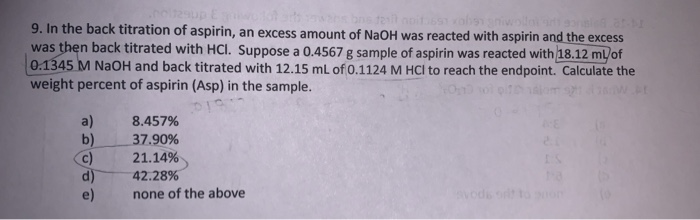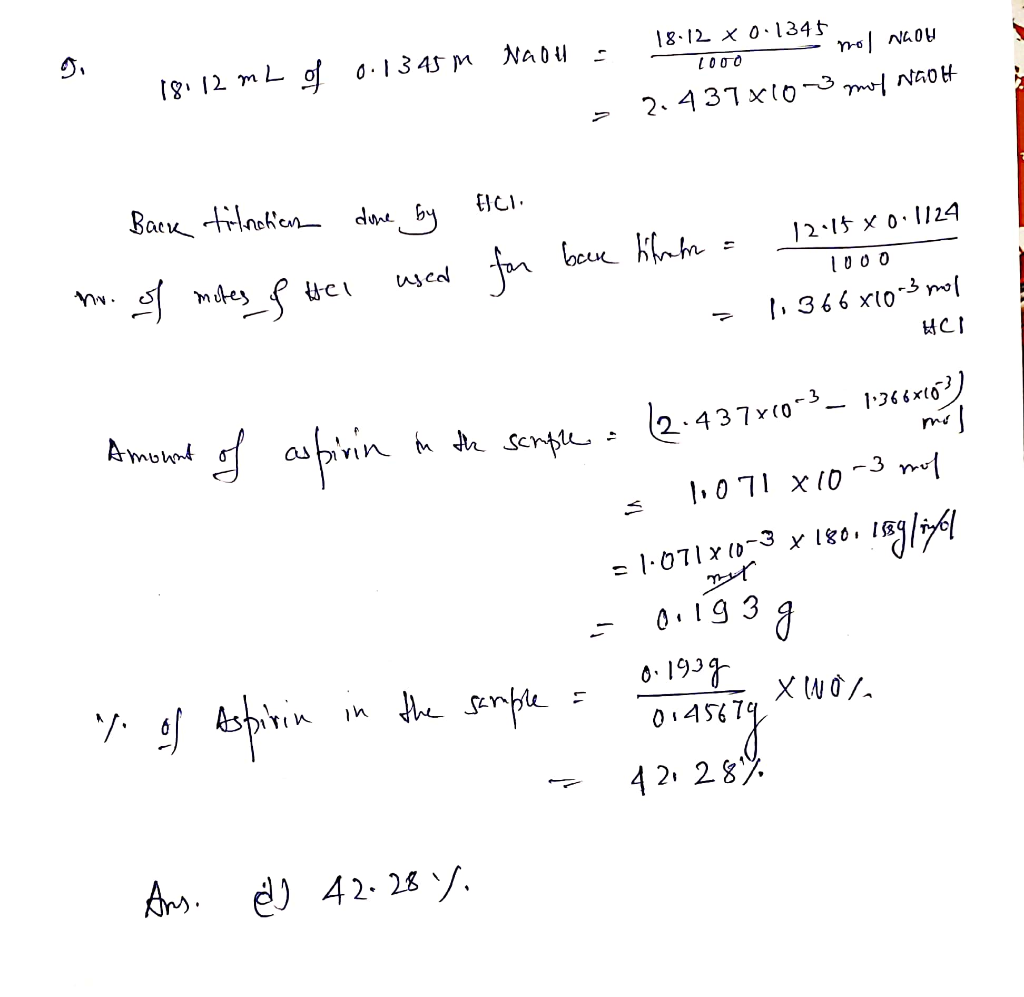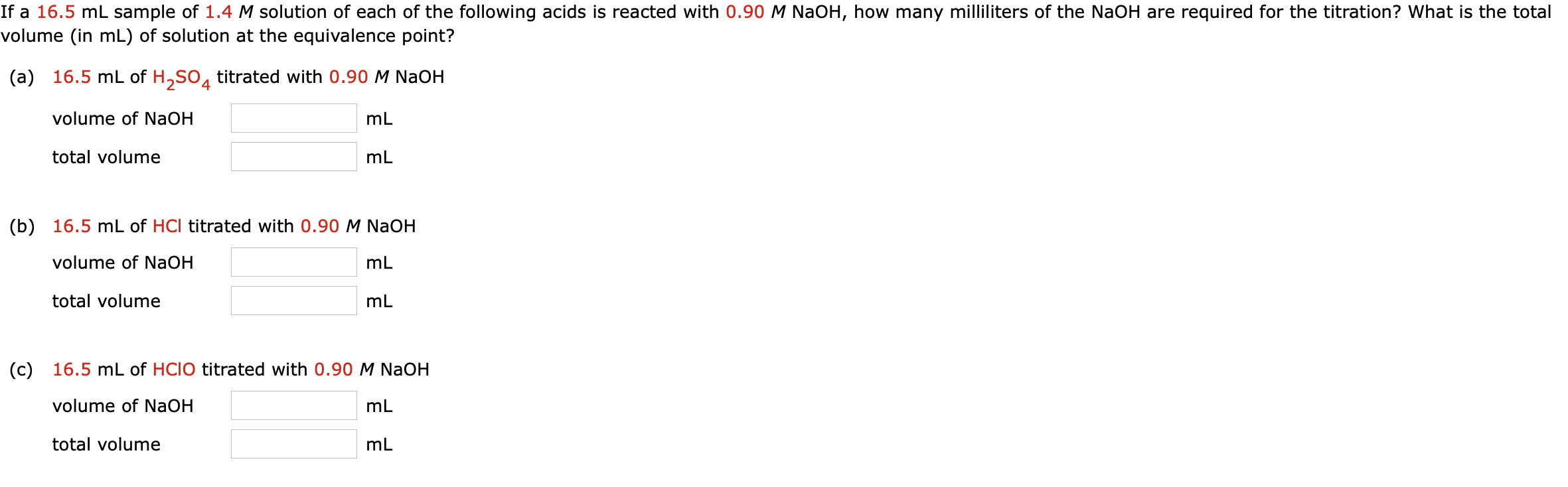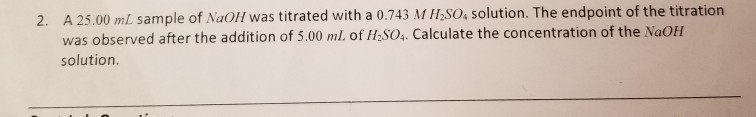# 9. In the back titration of aspirin, an excess amount of NaOH was reacted with aspirin...9. In the back titration of aspirin, an excess amount of NaOH was reacted with aspirin and the excess was then back titrated with HCl. Suppose a 0.4567 g sample of aspirin was reacted with 18.12 ml/of 0.1345 M NaOH and back titrated with 12.15 mL of 0.1124 M HCl to reach the endpoint. Calculate the weight percent of aspirin (Asp) in the sample. 8.457% 37.90% 21.14% 42.28% none of the abovebecause 1 mole of aspirin reacts with 2 moles of NaOH. For example, if the amount of consumed NaOH is 100 moles, the aspirin should be 50 moles.

source: Based on equation of aspirin with NaOH
• Amount of consumed NaOH = 2.437*10-3  -̶ 1.366*10-3  =  1.071*10-3 mol

• From equation, the amount of consumed NaOH is double the amount of aspirin (2 moles NaOH = 1 mole aspirin)

• So the amount of aspirin = 1.071*10-3 / 2 = 0.535*10-3

• gm aspirin = mole*mwt = 0.535*10-3 * 180.16 = 0.096 gm

• % aspirin = 0.096/0.4567 *100 = 21.12%

source: My knowledge
answered by: Eslam B. Elkaeed, PhD
##### Add Answer of: 9. In the back titration of aspirin, an excess amount of NaOH was reacted with aspirin...
Similar Homework Help Questions
• ### In the back titration of aspirin, an excess amount of NaOH was reacted with aspirin and...

In the back titration of aspirin, an excess amount of NaOH was reacted with aspirin and the excess was then back titrated with HCl. Suppose a 0.4567 g sample of aspirin was reacted with 18.12 mL of 0.1345 M NaOH and back titrated with 12.15 mL of 0.1124 M HCl to reach the endpoint. Calculate the weight of aspirin (Asp) in the sample.

• ### In Experiment 1, a student determined the aspirin content of a tablet by a back titration procedure. The weight of the s...

In Experiment 1, a student determined the aspirin content of a tablet by a back titration procedure. The weight of the student’s tablet was 1.5500 g. After crushing and dissolving the tablet, the student added 54.00 mL of 0.1000 M NaOH. The back titration of the excess NaOH required 18.00 mL of 0.2000 M HCl. How many moles of aspirin were in the tablet?

• ### Soda Ash Back Titration Problem

A 0.4016 g sample of impure sodium carbonate (soda ash) is dissolved in 50 mL of distilled water. Phenolphthalein was added and 11.40 mL of 0.09942 M HCL was requiredto reach the first end point. Excess volume of HCL was added according to the lab procedure (if x is the amount of acid needed to complete the first titration, youwill add a total of 2x + 10mL of acid to ensure excess). After boiling, the excess acid was titrated with...

• ### analysis of aspirin

Molarity of NaOH 0.1 Molarity of HCl 0.1 Mass of Aspirin 0.503 gramsVolume of NaOH required to neutralize all acid material 26.2 mLVolume of NaOH used in hydrolysis 45.7 mLVolume of HCl in back-titration 2.6 mLCalculate grams of acetylsalicylic acid in sample and percent of aspirin

• ### If a 16.5 mL sample of 1.4 M solution of each of the following acids is reacted with 0.90 M NaOH, how many milliliters...If a 16.5 mL sample of 1.4 M solution of each of the following acids is reacted with 0.90 M NaOH, how many milliliters of the NaOH are required for the titration? What is the total volume (in mL) of solution at the equivalence point? (a) 16.5 mL of H,So, titrated with 0.90 M NaOH volume of NaOH mL total volume mL (b) 16.5 mL of HCl titrated with 0.90 M NaOH volume of NaOH ml total volume ml (c)...

• ### Back Titration

0.60g of impure K2S2O8 (270.32 g/mol) was dissolved in 50.0 mL solution and treated with an excess of 50.00 mL of 0.0701 M Na2C2O4.The excess oxalate required 21.06 mL of 0.02880 M KMnO4 to reach the end point.a) What is the weight percent of K2S2O8 in the impure reagent (three sig. figs)? % by massRemember, the end point is only an estimate of the equivalence point. To obtain more accurate results, a blank is often analyzed to determine the difference...

• ### 1. A student carries out a back titration to determine the concentration of ammonium chloride in a solution. The student...

1. A student carries out a back titration to determine the concentration of ammonium chloride in a solution. The student collects 10.80 mL of the original NH4Cl solution and dilutes it to 250.0 mL (we will refer to this as the dilute NH4Cl solution). Then 25.00 mL of the dilute NH4Cl solution are transferred to an Erlenmeyer flask and 25.00 mL of 0.1963 M NaOH are added. Calculate the moles of NaOH added to this Erlenmeyer flask. 2. In the...

• ### titration/solution problem

A 0.6370-g sample of impure Ca(OH)2 is dissolved inenough water to make 31.90 mL of solution. 20.00 mL of theresulting solution is then titrated with0.2593-M HCl. What is thepercent purity of the calcium hydroxide if the titration requires28.68 mL of the acid to reach the endpoint?

• ### A 25.00 mL sample of NaOH was titrated with a 0.743 M H2sO, solution. The endpoint of the titration was observed aft...A 25.00 mL sample of NaOH was titrated with a 0.743 M H2sO, solution. The endpoint of the titration was observed after the addition of 5.00 ml. of H2SO4. Calculate the concentration of the NaOH 2. solution.

• ### weight percentageA 4.00 g sample of a granola bar was analyzed for its N content bythe Kjeldahl method, the liberated NH3 being collectedin 50.00 mL of 0.1448 M HCl.N NH3HCl + NH3 NH4+ + Cl-The excess HCl was back-titrated with 0.07374 M NaOH, requiring40.00 mL to reach the end point.HCl + NaOH NaCl+ H2OWhat is the weight percent N (14.01 g/mol) in the granola bar?

Free Homework App